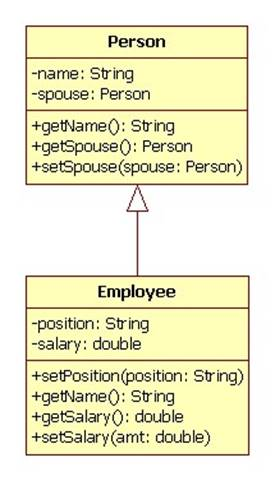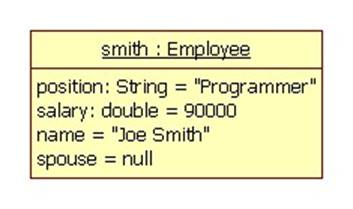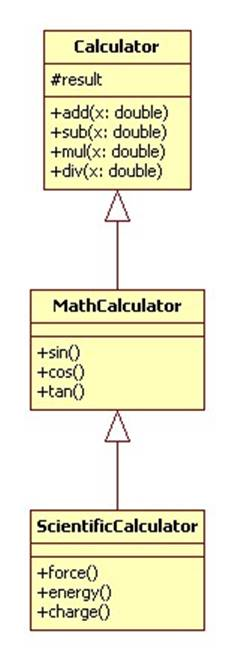# Generalization

What do we do when a library contains a class that is almost perfect? For example, the class has almost all of the methods we need, or it has all of the methods, but we are unhappy with the way some of the methods have been implemented?

One solution is to modify the class by adding the needed methods and replacing the unsatisfactory implementations. But this would be equivalent to writing in a library book.

Another solution is to start from scratch: create a new class that is almost identical to the existing class but with additional methods and new implementations of some existing methods. (Of course the class would need a new name or would need to be placed in a different package from the original class.)

This leads to code replication (not to mention work replication.) Code replication should be avoided. Not only is it wasteful, but what happens when the author of the original class finds and fixes a bug in one of the method implementations? How will this fix get propagated to all of the many copies of the method?

The right solution is to create a new class by extending the original class.

## Example

An HR application wants to represent employees as objects. A person class already exists in a library and is almost perfect:

class Person {
private String name;
private Person spouse = null;
public Person(String name) {
this.name = name;
}
public void setSpouse(Person spouse) {
this.spouse = spouse;
}
public Person getSpouse() { return spouse; }
public String getName() { return name; }
}

There are several small problems: employees have positions (e.g. "manager", "programmer", "CEO", etc.) which should always be appended to the name. Also, employees have salaries.

The solution is to create a new Employee class that extends the Person class. Here's the UML notation:

##The arrow connecting Employee to Person is called "generalization" because Person generalizes Employee. It is sometimes read as "is-a" as in "Every employee is-a person".

Here is the Java interpretation:

class Employee extends Person {
private String position;
private double salary;
public Employee(String name) {
super(name);
salary = 0;
position = null;
}
public void setPosition(String position) {
this.position = position;
}
public String getName() {
return super.getName() + ": " + position;
}
public double getSalary() { return salary; }
public void setSalary(double amt) {
salary = amt;
}
}

Notice that in UML and in Java we don't need to re-declare setSpouse and getSpouse. The methods and fields of Person will automatically be inherited by the Employee class.

Note the Employee constructor must initialize its inherited fields, but these fields are private. To get around this problem, call super.

## Creating Objects

Employee smith = new Employee("Joe Smith");
smith.setPosition("Programmer");
smith.setSalary(90000);

Here's an object icon:Here are some method invocations:

smith.getSpouse(); // returns null
smith.getName();   // returns "Joe Smith: Programmer"

## Subclasses

In the following diagram Employee extends Person, Programmer and Manager extend Employee. Programmer and Manager inherit all of the fields and methods of Employee, the declared methods and fields as well as the inherited.We say that A is a subclass of B (notation: A <: B) if A = B, A extends B, or if A extends C, where C is a subclass of B.

Person has four subclasses in this diagram.

Person <: Person
Employee <: Person
Programmer <: Employee <: Person => Programmer <: Person
Manager <: Employee <: Person => Manager <: Person

If A is a subclass of B, then A inherits all of the methods and fields of B.

Note that in Java all classes are subclasses of the Object class:

A <: Object

## Example

In the following example Calculator has three subclasses:In Java generalization translates into the extends relationship:

class MathCalculator extends Calculator {
public void sin() {
result = Math.sin(result);
}
// etc.
}

In C++ generalization translates into the derived class relationship:

class MathCalculator: public Calculator
{
public:
void sin()
{
result = sin(result);
}
// etc.
};

Note that MathCalculator can access the result member variable inherited from Calculator because it is protected instead of private.

A variable of type Calculator can contain a reference to a MathCalculator or an EngineeringCalculator:

Calculator calc = new ScientificCalculator();

This is made possible by inheritance: a subclass inherits the members of its super-class. For example, assume we create a Math Calculator:

MathCalculator mathCalc = new MathCalculator();

We can call all of the methods inherited from Calculator: